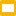# 24 Game in Google Slides

## Practice basic arithmetic, PEMDAS, build creativity

Subject: Math, Basic Operations, Mental Math
Grade Levels: 1st, 2nd, 3rd, 4th, 5th
Resource Type: Activities, Games, Google Apps
File Type: Google Slides™ (15 pages)
Practice basic arithmetic, PEMDAS, build creativity
To Use: Click on the gear icon and then click ‘Open editor’Make 24: Use only one number from each color group, and any operators +, -, x, /, and ( ) in Google Slides.

Practice basic arithmetic, PEMDAS, build creativity

Instructions:

→ Use exactly one number (1,2,3,4,5,6,7,8,9,10,11,12,13)
from each color group, and any operators +, -, x, /, and ( ),
to make an expression that equals 24.

→ Drag and drop the numbers and operators to the bottom bar

→ Go to the next slide and try to come up with another solution.

You can make your own version of the 24 game,
with different colors, fonts, sizes, and backgrounds.

Curriculum Standards:

CCSS
4.NBT.B.6
Find whole-number quotients and remainders with up to four-digit dividends and one-digit divisors, using strategies based on place value, the properties of operations, and/or the relationship between multiplication and division. Illustrate and explain the calculation by using equations, rectangular arrays, and/or area models.
CCSS
4.NBT.B.5
Multiply a whole number of up to four digits by a one-digit whole number, and multiply two two-digit numbers, using strategies based on place value and the properties of operations. Illustrate and explain the calculation by using equations, rectangular arrays, and/or area models.
CCSS
4.NBT.B.4
Fluently add and subtract multi-digit whole numbers using the standard algorithm.
CCSS
3.NBT.A.3
Multiply one-digit whole numbers by multiples of 10 in the range 10–90 (e.g., 9 × 80, 5 × 60) using strategies based on place value and properties of operations.
CCSS
3.NBT.A.2
Fluently add and subtract within 1000 using strategies and algorithms based on place value, properties of operations, and/or the relationship between addition and subtraction.
CCSS
2.NBT.B.9
Explain why addition and subtraction strategies work, using place value and the properties of operations.
CCSS
2.NBT.A.2
Count within 1000; skip-count by 5s, 10s, and 100s.
CCSS
1.NBT.C.5
Given a two-digit number, mentally find 10 more or 10 less than the number, without having to count; explain the reasoning used.
CCSS
1.NBT.C.4
Add within 100, including adding a two-digit number and a one-digit number, and adding a two-digit number and a multiple of 10, using concrete models or drawings and strategies based on place value, properties of operations, and/or the relationship between addition and subtraction; relate the strategy to a written method and explain the reasoning used. Understand that in adding two-digit numbers, one adds tens and tens, ones and ones; and sometimes it is necessary to compose a ten.